Author: Oscar Cronquist Article last updated on February 14, 2023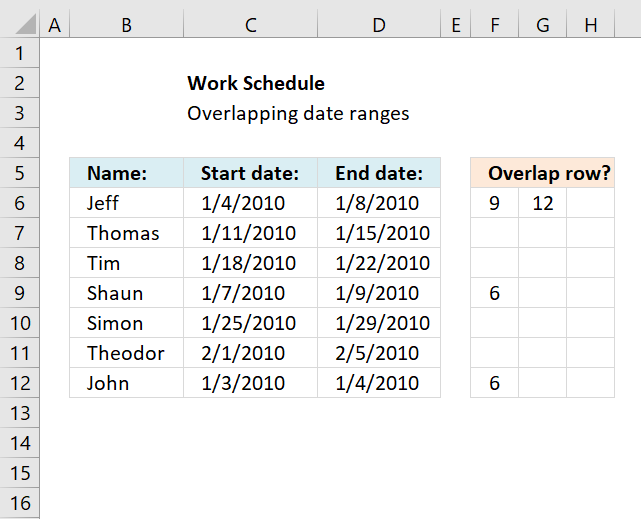This article demonstrates a formula that points out row numbers of records that overlap the current record based on a start and end date.

## 1. Identify rows of overlapping records - Excel 365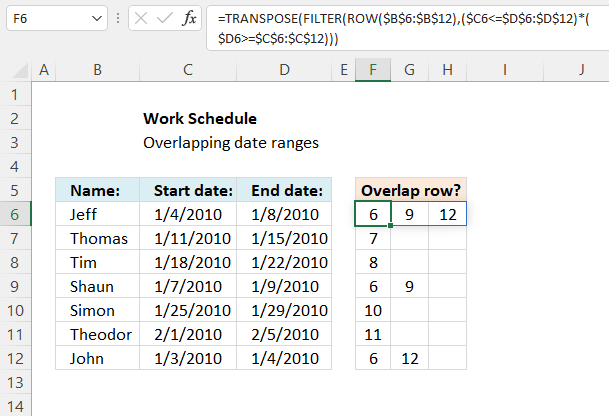The formula in cell F6 checks whether the date range specified on row 6 overlaps any of the remaining date ranges in cell range F6:D12 and then returns the corresponding row numbers. It always returns the current row number as a result of the date range always overlapping itself.

Excel 365 dynamic formula in cell F6:

=TRANSPOSE(FILTER(ROW(\$B\$6:\$B\$12), (\$C6<=\$D\$6:\$D\$12)*(\$D6>=\$C\$6:\$C\$12)))

### 1.1 Explaining formula

#### Step 1 - Calculate row numbers based on a cell reference

The ROW function calculates the row number of a cell reference.

Function syntax: ROW(reference)

ROW(\$B\$6:\$B\$12)

returns

{6;7;8;9;10;11;12}

#### Step 2 - Check if start date is smaller than or equal to all end dates

The less than and equal signs are logical operators that let you check if a number is smaller or equal to another number.

\$C6<=\$D\$6:\$D\$12

becomes

40182<={40186;40193;40200;40187;40207;40214;40182}

and returns

{TRUE; TRUE; TRUE; TRUE; TRUE; TRUE; TRUE}.

#### Step 3 - Check if end date is larger than or equal to all start dates

The larger than and equal signs are logical operators that let you check if a number is smaller or equal to another number.

\$D6>=\$C\$6:\$C\$12

becomes

40186>={40182;40189;40196;40185;40203;40210;40181}

and returns

{TRUE; FALSE; FALSE; TRUE; FALSE; FALSE; TRUE}.

#### Step 4 - Multiply arrays (AND logic)

The parentheses let you control the order of operation, we need to evaluate the logical operators before we multiply the arrays.

(\$C6<=\$D\$6:\$D\$12)*(\$D6>=\$C\$6:\$C\$12)

becomes

{TRUE; TRUE; TRUE; TRUE; TRUE; TRUE; TRUE}*{TRUE; FALSE; FALSE; TRUE; FALSE; FALSE; TRUE}

and returns

{1;0;0;1;0;0;1}.

Boolean values are converted to their numerical equivalents: 1 - TRUE , 0 (zero) - FALSE.

#### Step 5 - Filter row numbers based on an array containing boolean values

The FILTER function extracts values/rows based on a condition or criteria.

Function syntax: FILTER(array, include, [if_empty])

FILTER(ROW(\$B\$6:\$B\$12), (\$C6<=\$D\$6:\$D\$12)*(\$D6>=\$C\$6:\$C\$12))

becomes

FILTER({6;7;8;9;10;11;12},{1;0;0;1;0;0;1})

and returns {6; 9; 12}.

#### Step 6 - Rearrange vertical values horizontally

The TRANSPOSE function converts a vertical range to a horizontal range, or vice versa.

Function syntax: TRANSPOSE(array)

TRANSPOSE(FILTER(ROW(\$B\$6:\$B\$12), (\$C6<=\$D\$6:\$D\$12)*(\$D6>=\$C\$6:\$C\$12)))

## 2. Identify rows of overlapping records - earlier versionsHi Oscar,Great website! Keep up the good work.I have a question as to how to expand this to the next step. Per your initial example we can see that Jeff in Row 1 and Shaun in Row 4 overlap their work schedule. I would like to know if there were a way, say for instance in Range E6:E12, to display Jeff’s Row number next to Shaun’s name and visa – versa. I can see how this will easily display what record numbers overlap when there is only one overlap in the range. Can we differentiate the records when there are two overlaps? Let’s say Theodor in Row 6 also has an overlap with Thomas in Row 2.Now the data becomes confusing because we have to determine, from the 4 grayed rows, who overlaps with whom.Can a formula return the Row value of the “matching” overlap record?
In Jeff’s E6 cell it would indicate “Row 4” as to the matching record, and the reverse for Shaun. Shaun’s E9 cell would indicate “Row 1” as the matching record.
And at the same time Theodor’s E11 cell would reflect “Row 2” for Thomas’s record and Thomas’ E7 cell would show “Row 6” for Theodor.I haven’t even touched on the tougher one, such as what happens when John in Row 7 has an end date of 2010-01-07, thus overlapping with both Jeff and Shaun!One step at a time. :)Thanks

The array formula in cell F6 returns the rows of overlapping date ranges for the current record. The record on row 6 overlaps with both records on rows 9 and 12.

Records on row 9 and 12 show they overlap with the record on row 6, this makes it much easier to spot overlapping records.

Formula in cell F6:

=IFERROR(SMALL(IF((\$C6<=\$D\$6:\$D\$12)*(\$D6>=\$C\$6:\$C\$12), IF(ROW(\$C\$6:\$C\$12)=ROW(), "", ROW(\$C\$6:\$C\$12)), ""), COLUMNS(\$A\$1:A1)), "")

#### How to enter an array formula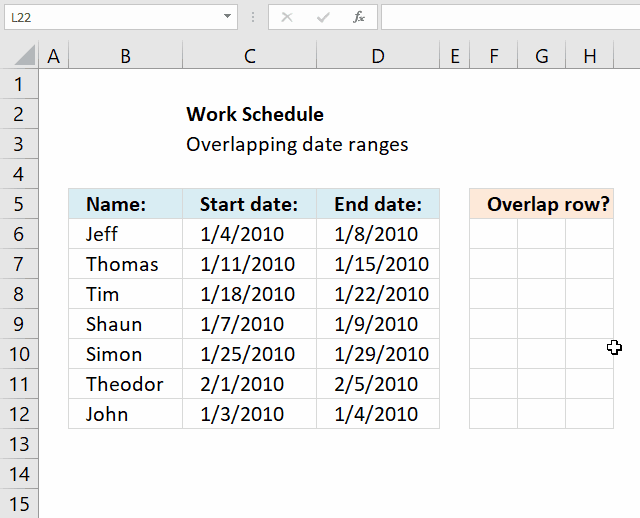You need to enter the formula in cell F6 as an array formula if you have an older Excel version than Excel 365 subscription.

1. Copy above array formula (Ctrl + c).
2. Double press with left mouse button on cell F6.
3. Paste above array formula (Ctrl + v).
4. Press and hold CTRL + SHIFT simultaneously.
5. Press Enter once.
6. Release all keys on keyboard.

The formula now has a beginning and ending curly bracket, like this: {=array_formula}

How to copy array formula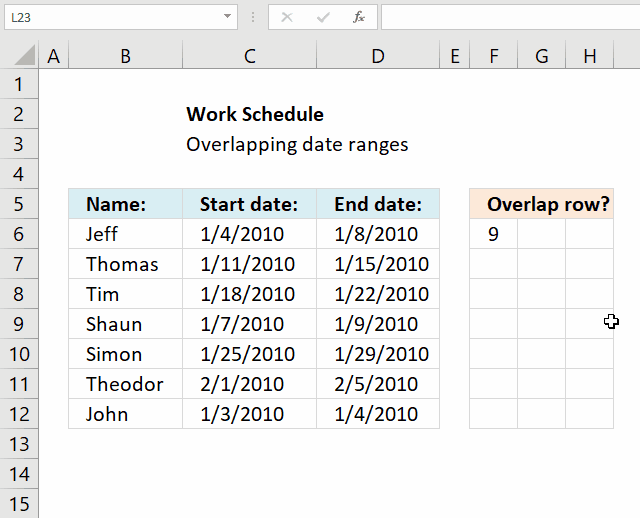1. Select cell F6.
2. Press and hold left mouse button on the green dot located at the bottom right corner of the selected cell.
3. Drag with the mouse as far as needed to the right.
4. Press and hold left mouse button on the green dot located at the bottom right corner of the selected cell range.
5. Drag with the mouse downwards as far as needed.

The formula contains relative cell references, they change when the cell is copied. You need to copy cells to get this to work.

### Concatenated row numbers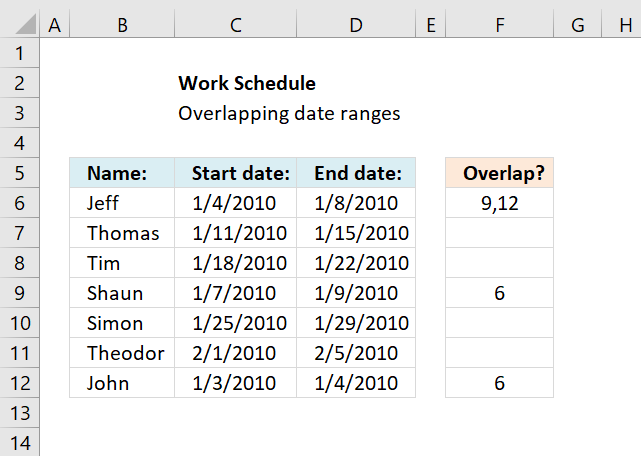If you rather have row numbers concatenated in a single cell use the following formula.

=TEXTJOIN(", ", TRUE, IF((\$C6<=\$D\$6:\$D\$12)*(\$D6>=\$C\$6:\$C\$12), IF(ROW(\$C\$6:\$C\$12)=ROW(), "", ROW(\$C\$6:\$C\$12)), ""))

The TEXTJOIN function is available for Excel 365 subscribers, there is also User Defined Function (UDF) on the same webpage if you own an earlier version of Excel.

### Explaining formula in cell F6I recommend using the "Evaluate Formula" tool located on the Formula tab on the ribbon. Press with left mouse button on the "Evaluate Formula" button, a dialog box appears, see image above.

Press with left mouse button on the Evaluate button to see next calculation step.

#### Step 1 - Identify overlapping date ranges

The logical operators <> and = allow you to compare dates and find overlapping date ranges.

(\$B6<=\$C\$6:\$C\$12)*(\$C6>=\$B\$6:\$B\$12)

becomes

({TRUE; TRUE; TRUE; TRUE; TRUE; TRUE; TRUE})*({TRUE; FALSE; FALSE; TRUE; FALSE; FALSE; TRUE})

becomes

{1;0;0;1;0;0;1}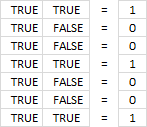If you multiply boolean values you get this in Excel:

• TRUE * TRUE = 1
• TRUE * FALSE = 0
• FALSE *FALSE = 0

This is AND logic and the asterisk allows you to multiply arrays row-wise.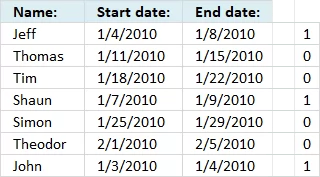If you use the AND function it will apply AND logic to all values and return a single value, that is the reason we can't use it here.

We want it to return an array so we can easily identify the overlapping date ranges.

The array has the same number of values as there are date ranges, the position in the array corresponds to the position in the list of records.

#### Step 2 - Create an array containing row numbers except for the current row number

To be able to return the correct row numbers we must create an array that is equally large as the previous array.

We don't want to return the date range for the current record so we need to figure out a way to remove the current row number from the array.

IF(ROW(\$C\$6:\$C\$12)=ROW(), "", ROW(\$C\$6:\$C\$12)), "")

becomes

IF({6;7;8;9;10;11;12}=6, "", {6;7;8;9;10;11;12}, "")

returns

{"";7;8;9;10;11;12}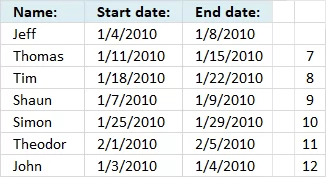#### Step 3 - If current date range overlaps another date range then return row number

The logical expressions we built in step 1 is now used in an IF function to extract the correct row numbers of date ranges that overlap.

IF((\$B6<=\$C\$6:\$C\$12)*(\$C6>=\$B\$6:\$B\$12), IF(ROW(\$B\$6:\$B\$12)=ROW(), "", ROW(\$B\$6:\$B\$12)), "")

becomes

IF({1;0;0;1;0;0;1}, {"";7;8;9;10;11;12}, "")

returns

{"";"";"";9;"";"";12}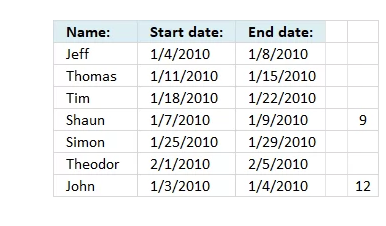#### Step 4 - Find the k-th smallest row number

The last step is to extract the smallest number based on where in worksheet the formula is calculated.

SMALL(IF((\$B6<=\$C\$6:\$C\$12)*(\$C6>=\$B\$6:\$B\$12), IF(ROW(\$B\$6:\$B\$12)=ROW(), "", ROW(\$B\$6:\$B\$12)), ""),COLUMN(A1))

becomes

SMALL({"";"";"";9;"";"";12}, COLUMN(A1))

The COLUMN function calculates the column number based on a cell reference. The cell reference A1 is relative meaning it changes when the cell is copied to cells to the right.

SMALL({"";"";"";9;"";"";12}, COLUMN(A1))

becomes

SMALL({"";"";"";9;"";"";12}, 1)

and returns 9 in cell F6.

In cell G6 the formula changes to

SMALL({"";"";"";9;"";"";12}, COLUMN(B1))

and becomes

SMALL({"";"";"";9;"";"";12}, 2)

and returns 12 in cell G6.### Get the Excel fileIdentify-overlapping-date-rangesv3.xlsx

### Recommended articles

How to find overlapping date/time ranges in Excel?

Check if two ranges of dates overlap (Excel Formulas)